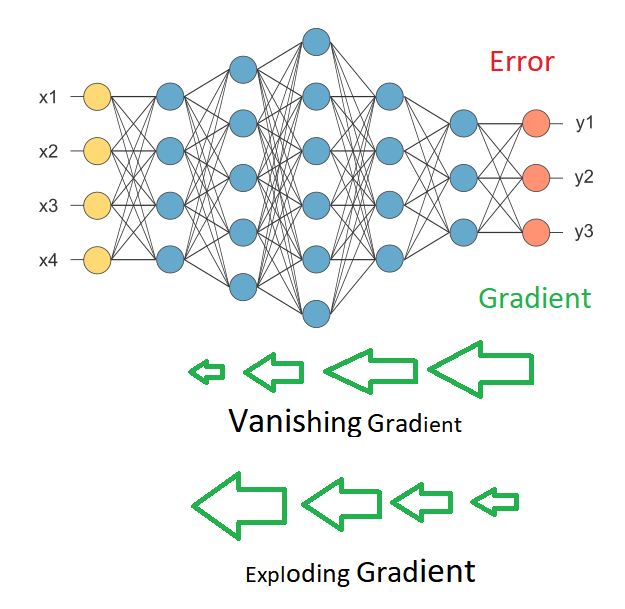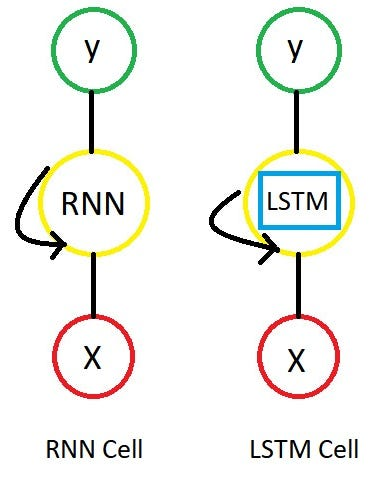# Chapter 10.1: DeepNLP — LSTM (Long Short Term Memory) Networks with Math.

Note: I am writing this article with the assumption that you know the deep learning a bit. In case if you don’t know much, Please read my earlier stories to understand the entire series on deep learning.

In the last story we talked about Recurrent neural networks, so we now know what RNN’s are, How they work and what kind of problems it can solve and also we talked about a limitation in RNN’s which is

Vanishing /exploding gradient problem

We all know that a neural network uses an algorithm called BackPropagation to update the weights of the network. So what BP does is

It first calculates the gradients from the error using the chain rule in Calculus, then it updates the weights(Gradient descent).

since the BP starts from the output layer to all the way back to input layer , In a simple neural network we may not face problems with updating weights but in a deep neural network we might face some issues.Deep NN

As we go back with the gradients , It is possible that the values get either smaller exponentially which causes Vanishing Gradient problem or larger exponentially which causes Exploding Gradient problem.

Due to this we get the problems of training the network.

In RNN’s, we have time steps and current time step value depends on the previous time step so we need to go all the way back to make an update.

There are couple of remedies there to avoid this problem.

We can use ReLu unit as an activation function, RMS Prop as an optimization algorithm and LSTM’s or GRU’s.

so Lets focus on LSTM

LSTM ( Long Short Term Memory ) Networks are called fancy recurrent neural networks with some additional features.Rolled Network

Just like RNN, we have time steps in LSTM but we have extra piece of information which is called “MEMORY” in LSTM cell for every time step.

So the LSTM cell contains the following components

1. Forget Gate “f” ( a neural network with sigmoid)
2. Candidate layer “C`"(a NN with Tanh)
3. Input Gate “I” ( a NN with sigmoid )
4. Output Gate “O”( a NN with sigmoid)
5. Hidden state “H” ( a vector )
6. Memory state “C” ( a vector)

Here is the diagram for LSTM cell at the time step t

Don’t panic I will explain every single hecking detail of it. Just get the overall picture stored in your brain.

Lemme take only one time step (t) and explain it.

What are the inputs and outputs of the LSTM cell at any step ??

Inputs to the LSTM cell at any step are X (current input) , H ( previous hidden state ) and C ( previous memory state)

Outputs from the LSTM cell are H ( current hidden state ) and C ( current memory state)

Here is the diagram for a LSTM cell at T time step.

How does the LSTM flow work??

If you observe carefully,the above diagram explains it all.

Anyway, lemme also try with words

Forget gate(f) , Cndate(C`), Input gate(I), Output Gate(O)

are single layered neural networks with the Sigmoid activation function except candidate layer( it takes Tanh as activation function)

These gates first take input vector.dot(U) and previous hidden state.dot(W) then concatenate them and apply activation function

finally these gate produce vectors ( between 0 and 1 for Sigmoid, -1 to 1 for Tanh) so we get four vectors f, C`, I, O for every time step.

Now let me tell you an important piece called Memory state C

This is the state where the memory (context) of input is stored

Ex : Mady walks in to the room, Monica also walks in to the room. Mady Said “hi” to ____??

Inorder to predict correctly Here it stores “Monica” into memory C.

This state can be modified. I mean LSTM cell can add /remove the information.

Ex : Mady and Monica walk in to the room together , later Richard walks in to the room. Mady Said “hi” to ____??

The assumption I am making is memory might change from Monica to Richard.

I hope you get the idea.

so LSTM cell takes the previous memory state Ct-1 and does element wise multiplication with forget gate (f)

Ct = Ct-1*ft

if forget gate value is 0 then previous memory state is completely forgotten

if forget gate value is 1 then previous memory state is completely passed to the cell ( Remember f gate gives values between 0 and 1 )

Now with current memory state Ct we calculate new memory state from input state and C layer.

Ct= Ct + (It*C`t)

Ct = Current memory state at time step t. and it gets passed to next time step.

Here is flow diagram for Ct

Finally, we need to calculate what we’re going to output. This output will be based on our cell state Ct but will be a filtered version. so we apply Tanh to Ct then we do element wise multiplication with the output gate O, That will be our current hidden state Ht

Ht = Tanh(Ct)

We pass these two Ct and Ht to the next time step and repeat the same process.

Here is the full diagram for LSTM for different time steps.

Well I hope you get the idea of LSTM.

### Conclusion

RNN’ s have been an active research area and many people have been achieving amazing results lately using RNN’s (most of all are using LSTMs) They really work a lot better for most tasks!

LSTM’s are really good but still face some issues for some problems so many people developed other methods also after LSTM’s ( hope I can cover later stories)

So That’s it for this story , In the next story I will be writing about more advanced topics. Have a great day….!

Suggestions /questions are welcome.

Photos are designed using Paint in windows inspired by Christopher Olah Understanding LSTMs;

See ya!